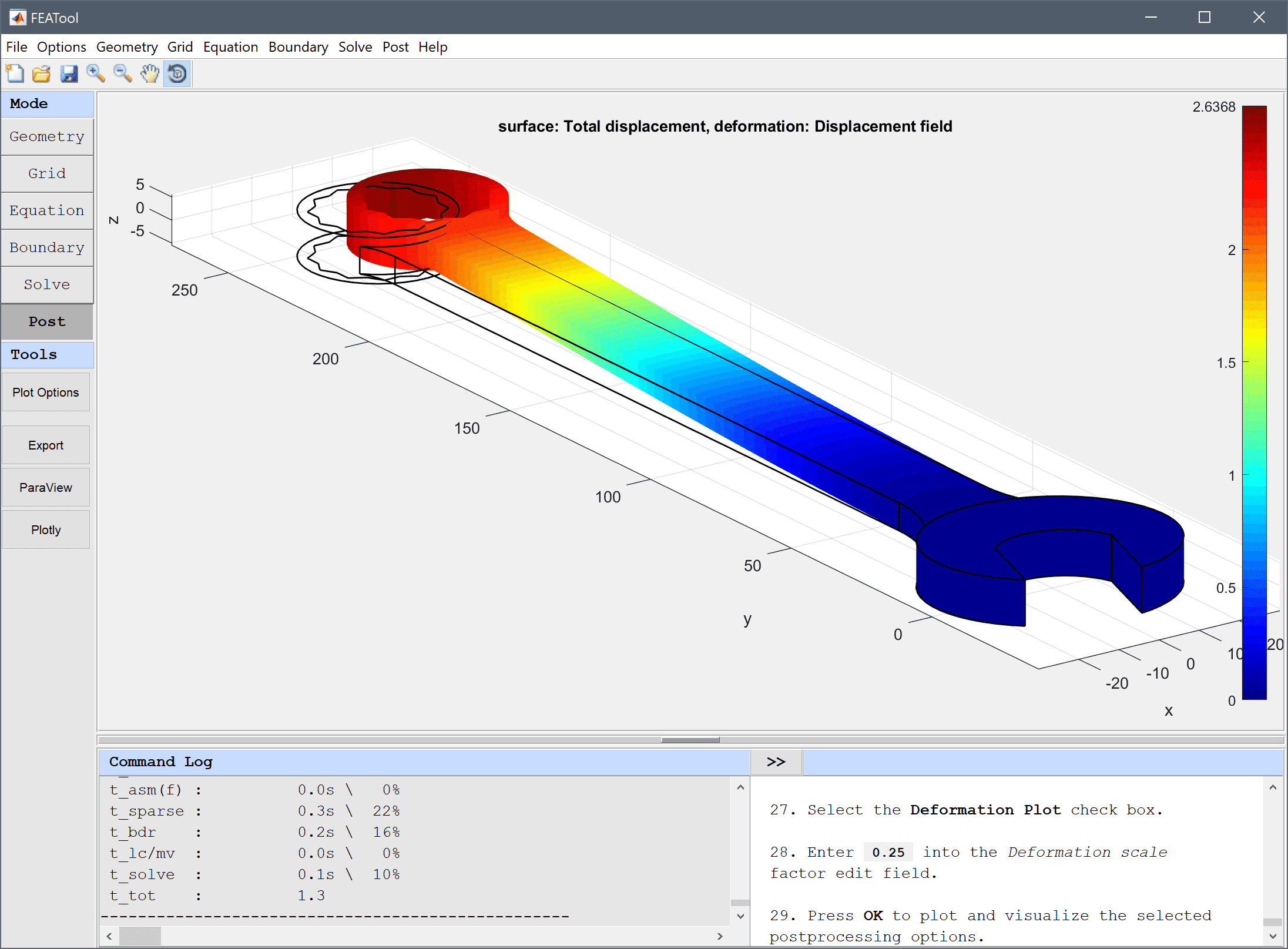FEATool Multiphysics  v1.15.5 Finite Element Analysis Toolbox
Deformation of a Spanner

Tutorial example of calculating deformation and deflection in a spanner where the geometry is given in and imported from a STL CAD file.# Tutorial

This model is available as an automated tutorial by selecting Model Examples and Tutorials... > Structural Mechanics > Deformation of a Spanner from the File menu. Or alternatively, follow the step-by-step instructions below.

1. To start a new model click the New Model toolbar button, or select New Model... from the File menu.
2. Select the 3D radio button.
3. Select the Linear Elasticity physics mode from the Select Physics drop-down menu.
4. Press OK to finish the physics mode selection.

The spanner geometry will be imported from an STL file Instead of creating it with the built-in CAD tools.

1. Select Import Geometry > STL Format.. from the Geometry menu, and choose the spanner1.stl file to import.
2. The STL file has been prepared with each boundary in a separate STL section. Automatic boundary detection is therefore not required, uncheck the corresponding checkbox to disable this. Leave the other settings at their default values and press OK to import the geometry.
3. Switch to Grid mode by clicking on the corresponding Mode Toolbar button.
4. Press the Settings Toolbar button.

The built-in grid generator does not handle faceted/STL geometries robustly, so it is recommended to use either the Robust or Gmsh grid generators for these types of geometries.

1. Select robust from the Grid generation algorithm drop-down menu.
2. Enter 5 into the Subdomain Grid Size edit field.
3. Press the Generate button to call the grid generation algorithm.
4. Press OK to finish and close the dialog box.
5. Switch to Equation mode by clicking on the corresponding Mode Toolbar button.
6. Equation and material coefficients can be specified in Equation/Subdomain mode. In the Equation Settings dialog box that automatically opens, enter 0.29 for the Poisson's ratio and 190e3 for the Modulus of elasticity. The other coefficients can be left to their default values. Press OK to finish the equation and subdomain settings specification.

Note that FEATool works with any unit system, and it is up to the user to use consistent units for geometry dimensions, material, equation, and boundary coefficients. Here the geometry scale is in mm and thus the units of the equation coefficients have been adjusted accordingly.

1. Switch to Boundary mode by clicking on the corresponding Mode Toolbar button.

Fix the displacement in all directions for the jaws of the spanner.

1. Select 13 and 14 in the Boundaries list box.
2. Select the Fixed displacement, w radio button.
3. Select the Fixed displacement, v radio button.
4. Select the Fixed displacement, u radio button.

Enter the equivalent load expression F/A*(y>140) on one of the side boundaries. The switch expression ensures that the load is only active and applied on the top section where y is larger than 140 mm.

1. Select 3 in the Boundaries list box.
2. Enter 1000/(6*80)*(y>140) into the Displacement/load, x-component edit field.
3. Press OK to finish the boundary condition specification.
4. Switch to Solve mode by clicking on the corresponding Mode Toolbar button.
5. Press the = Toolbar button to call the solver. After the problem has been solved FEATool will automatically switch to postprocessing mode and plot the computed solution.

Plot the displacement and verify that the maximum displacement is around 2.5 mm.

1. Press the Plot Options Toolbar button.
2. Select Total displacement from the Predefined surface plot expressions drop-down menu.
3. Select the Deformation Plot check box.
4. Press OK to plot and visualize the selected postprocessing options.

The deformation of a spanner structural mechanics model has now been completed and can be saved as a binary (.fea) model file, or exported as a programmable MATLAB m-script text file (available as the example ex_spanner script file), or GUI script (.fes) file.